# Precalculus : Solve a System of Equations in Three Variables Using Augmented Matrices

## Example Questions

### Example Question #1 : Solve A System Of Equations In Three Variables Using Augmented Matrices

Express this system of equations as an augmented matrix: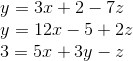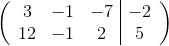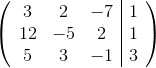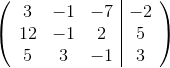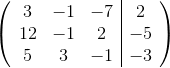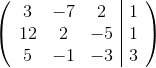Explanation:

Arrange the equations into the form: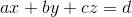, where a,b,c,d are constants.

Then we have the system of equations: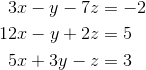.

The augmented matrix is found by copying the constants into the respective rows and columns of a matrix.

The vertical line in the matrix is analogous to the = sign thus resulting in the following:### Example Question #2 : Solve A System Of Equations In Three Variables Using Augmented Matrices

Using an augmented matrix, solve the following system of equations: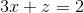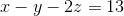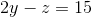Which of the following are the values of,,andthat satisfy this system?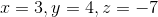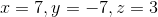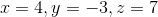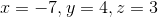Explanation:

Create an augmented matrix by entering the coefficients into one matrix and appending a vector to that matrix with the constants that the equations are equal to. Then you can row reduce to solve the system.

First, lets make this augmented matrix: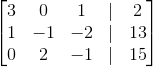Now we can row reduce the matrix using the three row reduction operations: mutliply a row, add one row to another, swap row positions.

First, we can subtract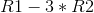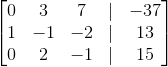Swap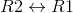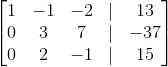Subtract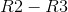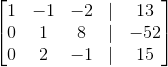Add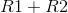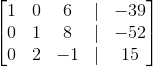Subtract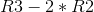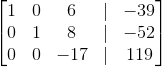Mulitply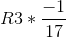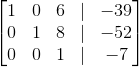You can stop here given that this augmented matrix can be rewritten as a system again with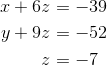, or you can continue using the matrix, subtracting multiples of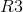from the other two rows to get an identity matrix yielding the solution to the system.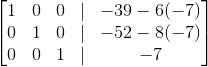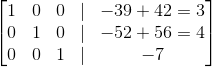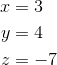### All Precalculus Resources